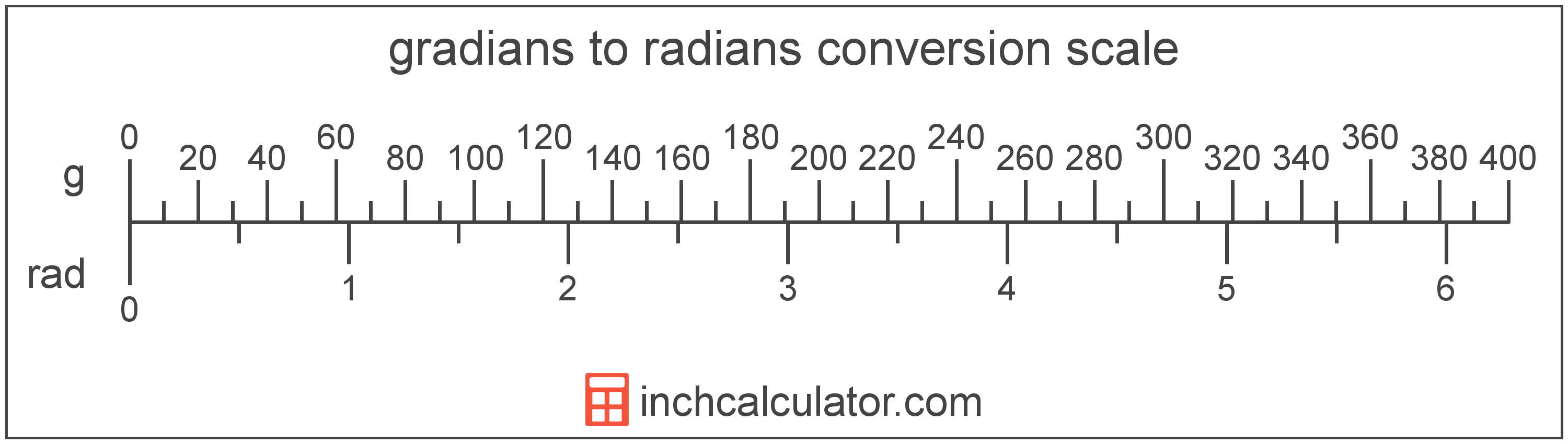Enter the angle in gradians below to get the value converted to radians.Since one gradian is equal to 0.015708 radians, you can use this simple formula to convert:

The angle in radians is equal to the angle in gradians multiplied by 0.015708.

For example, here's how to convert 5 gradians to radians using the formula above.A gradian is equal to 1/400 of a revolution or circle, or 9/10°. The grad, or gon, is more precisely defined as π/200, or 1.570796 × 10-2 radians.

This unit simplifies the measurements of right angles, as 90° is equal to 100 gradians.

A gradian is sometimes also referred to as a grad, gon, or grade. Gradians can be abbreviated as g, and are also sometimes abbreviated as gr or grd. For example, 1 gradian can be written as 1g, 1 gr, or 1 grd.

In the expressions of units, the slash, or solidus (/), is used to express a change in one or more units relative to a change in one or more other units.

A radian is the measurement of angle equal to the length of an arc divided by the radius of the circle or arc. 1 radian is equal to 180/π degrees, or about 57.29578°. There are about 6.28318 radians in a circle.

The radian is the SI derived unit for angle in the metric system. Radians can be abbreviated as rad, and are also sometimes abbreviated as c, r, or R. For example, 1 radian can be written as 1 rad, 1 c, 1 r, or 1 R.

Radians are often expressed using their definition. The formula to find an angle in radians is θ = s/r, where the angle in radians θ is equal to the arc length s divided by the radius r. Thus, radians may also be expressed as the formula of arc length over the radius.

Radians are also considered to be a "unitless" unit. That is, when multiplying or dividing by radians, the result does not include radians as part of the final units.

For example, when determining the length of an arc for a given angle, we use the formula above, rearranged to be s = θr. If θ is in radians and r is in meters, then the units of s will be meters, not radian-meters. If θ were in degrees, however, then s would have units of degree-meters.